www.batmath.it

Exercises on linear systems

1. Solve the system: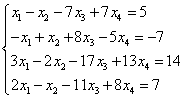.
2. Solve the system: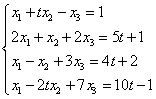, where t is a real number.
3. Reduce the matrix to reduced row-echelon form: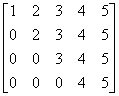.
4. Solve the homogeneous system: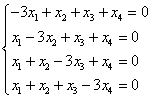.
5. For which values of t the following homogeneous system has a non trivial solution?.
6. Find a condition on the real numbers a, b, c, d under which the following matrix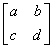is row-equivalent to the matrix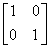.
7. Solve the system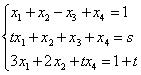, where t and s are real numbers.
8. Solve the system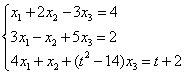, where t is a real number.
first published on march 15 2002 - last updated on september 01 2003